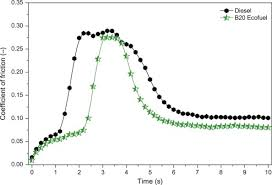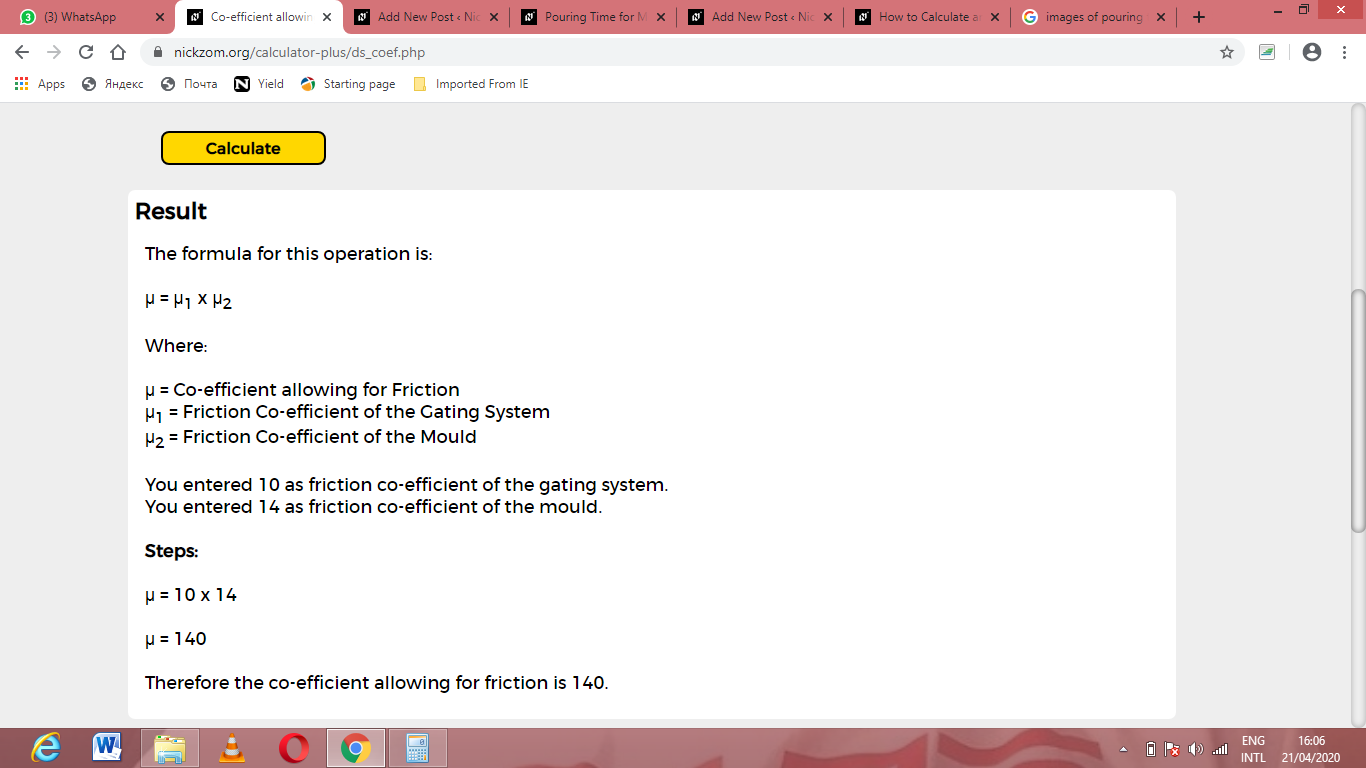# How to Calculate and Solve for Co-efficient allowing for Friction | Design of Gating SystemThe image above represents co-efficient allowing for friction.

To compute for co-efficient allowing for friction, two essential parameters are needed and these parameters are Friction Co-efficient of the Gating System (μ1) and Friction Co-efficient of the Mould (μ2).

The formula for calculating co-efficient allowing for friction:

μ = μ1 x μ2

Where:

μ = Co-efficient allowing for Friction
μ1 = Friction Co-efficient of the Gating System
μ2 = Friction Co-efficient of the Mould

Let’s solve an example;
Find the co-efficient allowing for friction when the friction co-efficient of the gating system is 10 and the friction co-efficient of the mould is 14.

This implies that;

μ1 = Friction Co-efficient of the Gating System = 10
μ2 = Friction Co-efficient of the Mould = 14

μ = μ1 x μ2
μ = 10 x 14
μ = 140

Therefore, the co-efficient allowing for friction is 140.

Calculating the Friction Co-efficient of the Gating System when the Co-efficient Allowing for Friction and the Friction Co-efficient of the Mould is Given.

μ1 = μ / μ2

Where;

μ1 = Friction Co-efficient of the Gating System
μ = Co-efficient allowing for Friction
μ2 = Friction Co-efficient of the Mould

Let’s solve an example;
Find the friction co-efficient of the gating system when the co-efficient allowing for friction is 45 and the friction co-efficient of the mould is 15.

This implies that;

μ = Co-efficient allowing for Friction – 45
μ2 = Friction Co-efficient of the Mould = 15

μ1 = μ / μ2
μ1 = 45 / 15
μ1 = 3

Therefore, the friction co-efficient of the gating system is 3.

Calculating the Friction Co-efficient of the Mould when the Co-efficient Allowing for Friction and the Friction Co-efficient of the Gating System is Given.

μ2 = μ / μ1

Where;

μ2 = Friction Co-efficient of the Mould
μ = Co-efficient allowing for Friction
μ1 = Friction Co-efficient of the Gating System

Let’s solve an example;
Find the friction co-efficient of the mould when the co-efficient allowing for friction is 50 and the friction co-efficient of the gating system is 25.

This implies that;

μ = Co-efficient allowing for Friction = 50
μ1 = Friction Co-efficient of the Gating System = 25

μ2 = μ / μ1
μ2 = 50 / 25
μ2 = 2

Therefore, the friction co-efficient of the mould is 2.

Nickzom Calculator – The Calculator Encyclopedia is capable of calculating the co-efficient allowing for friction.

To get the answer and workings of the co-efficient allowing for friction using the Nickzom Calculator – The Calculator Encyclopedia. First, you need to obtain the app.

You can get this app via any of these means:

To get access to the professional version via web, you need to register and subscribe for NGN 1,500 per annum to have utter access to all functionalities.
You can also try the demo version via https://www.nickzom.org/calculator

Apple (Paid) – https://itunes.apple.com/us/app/nickzom-calculator/id1331162702?mt=8
Once, you have obtained the calculator encyclopedia app, proceed to the Calculator Map, then click on Materials & Metallurgical under Engineering.Now, Click on Foundry Technology under Material & MetallurgicalNow, Click on Design of Gating System under Foundry TechnologyNow, Click on Co-efficient Allowing for Friction under Design of Gating SystemThe screenshot below displays the page or activity to enter your values, to get the answer for the co-efficient allowing for friction according to the respective parameters which are the Friction Co-efficient of the Gating System (μ1) and Friction Co-efficient of the Mould (μ2).Now, enter the values appropriately and accordingly for the parameters as required by the Friction Co-efficient of the Gating System (μ1) is 10 and Friction Co-efficient of the Mould (μ2) is 14.Finally, Click on CalculateAs you can see from the screenshot above, Nickzom Calculator– The Calculator Encyclopedia solves for the co-efficient allowing for friction and presents the formula, workings and steps too.